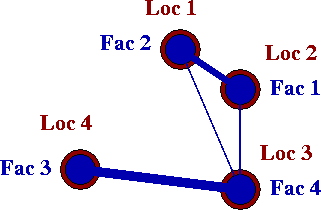The objective of the Quadratic Assignment Problem (QAP) is to assign $$n$$ facilities to $$n$$ locations in such a way as to minimize the assignment cost. The assignment cost is the sum, over all pairs, of the flow between a pair of facilities multiplied by the distance between their assigned locations.

## Problem Statement

The quadratic assignment problem (QAP) was introduced by Koopmans and Beckman in 1957 in the context of locating “indivisible economic activities”. The objective of the problem is to assign a set of facilities to a set of locations in such a way as to minimize the total assignment cost. The assignment cost for a pair of facilities is a function of the flow between the facilities and the distance between the locations of the facilities.

Consider a facility location problem with four facilities (and four locations). One possible assignment is shown in the figure below: facility 2 is assigned to location 1, facility 1 is assigned to location 2, facility 4 is assigned to location 3, and facility 4 is assigned to location 3. This assignment can be written as the permutation $$p = \{2, 1, 4, 3\}$$, which means that facility 2 is assigned to location 1, facility 1 is assigned to location 2, facility 4 is assigned to location 3, and facility 4 is assigned to location 3. In the figure, the line between a pair of facilities indicates that there is required flow between the facilities, and the thickness of the line increases with the value of the flow.To calculate the assignment cost of the permutation, the required flows between facilities and the distances between locations are needed.

Flows between facilities
$\begin{array}{|c|c|c|} \text{facility } i & \text{facility } j & \text{flow}(i,j) \\ \hline \hline 1 & 2 & 3 \\ 1 & 4 & 2 \\ 2 & 4 & 1 \\ 3 & 4 & 4 \end{array}$

Distances between locations
$$\begin{array}{|c|c|c|} \text{location } i & \text{location } j & \text{distance}(i,j) \\ \hline 1 & 3 & 53 \\ 2 & 1 & 22 \\ 2 & 3 & 40 \\ 3 & 4 & 55 \end{array}$$

Then, the assignment cost of the permutation can be computed as $$f(1,2) \cdot d(2,1) + f(1,4) \cdot d(2,3) + f(2,4) \cdot d(1,3) + f(3,4) \cdot d(3,4)$$ = $$3 \cdot 22 + 2 \cdot 40 + 1 \cdot 53 + 4 \cdot 55$$ = 419. Note that this permutation is not the optimal solution.

## Mathematical Formulation

Here we present the Koopmans-Beckmann formulation of the QAP. Given a set of facilities and locations along with the flows between facilities and the distances between locations, the objective of the Quadratic Assignment Problem is to assign each facility to a location in such a way as to minimize the total cost.

Sets
$$N = \{1, 2, \cdots, n\}$$
$$S_n = \phi: N \rightarrow N$$ is the set of all permutations

Parameters
$$F = (f_{ij})$$ is an $$n \times n$$ matrix where $$f_{ij}$$ is the required flow between facilities $$i$$ and $$j$$
$$D = (d_{ij})$$ is an $$n \times n$$ matrix where $$d_{ij}$$ is the distance between locations $$i$$ and $$j$$

Optimization Problem
$$\text{min}_{\phi \in S_n} \sum_{i=1}^n \sum_{j=1}^n f_{ij} \cdot d_{\phi(i) \phi(j)}$$

The assignment of facilities to locations is represented by a permutation $$\phi$$, where $$\phi(i)$$ is the location to which facility $$i$$ is assigned. Each individual product $$f_{ij} \cdot d_{\phi(i) \phi(j)}$$ is the cost of assigning facility $$i$$ to location $$\phi(i)$$ and facility $$j$$ to location $$\phi(j)$$.

## Solve some QAPs!

Follow the links below to test your skill at finding good solutions to QAPs of various sizes. Notice that as the problem size increases, it becomes much more difficult to find an optimal solution. As $$n$$ increases beyond a small number, it becomes impossible to enumerate and evaluate all possible assignment vectors. Instead, advanced solution algorithms are required to solve larger instances.

### QAP of size 4

Assign each facility (1, 2, 3, 4) to one location (A, B, C, D).

### QAP of size 5

Assign each facility (1, 2, 3, 4, 5) to one location (A, B, C, D, E).

### QAP of size 6

Assign each facility (1, 2, 3, 4, 5, 6) to one location (A, B, C, D, E, F).

### QAP of size 7

Assign each facility (1, 2, 3, 4, 5, 6, 7) to one location (A, B, C, D, E, F, G).

### QAP of size 8

Assign each facility (1, 2, 3, 4, 5, 6, 7, 8) to one location (A, B, C, D, E, F, G, H).

### QAP of size 9

Assign each facility (1, 2, 3, 4, 5, 6, 7, 8, 9) to one location (A, B, C, D, E, F, G, H, I)

## References

• Anstreicher, K.M. 2003. Recent advances in the solution of quadratic assignment problems. Mathematical Programming Series B 97, 27 - 42.
• Çela, E. 1998. The Quadratic Assignment Problem: Theory and Algorithms. Kluwer Academic Publishers, Dordrecht.
• Koopmans, T. C. and M. J. Beckmann. 1957. Assignment problems and the location of economic activities. Econometrica 25, 53 - 76.